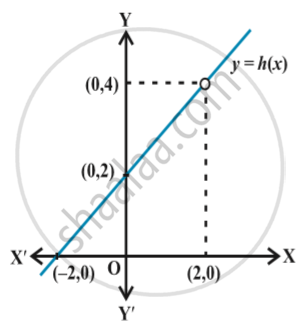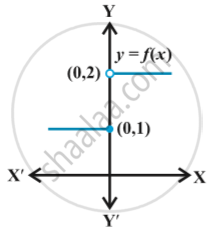# Introduction of Limits

#### notes

The function f(x) = x^2. Observe that as x takes values very close to 0, the value of f(x) also moves towards 0 .
lim_(x->0) f(x) = 0
(to be read as limit of f (x) as x tends to zero equals zero). The limit of f (x) as x tends to zero is to be thought of as the value f (x) should assume at x = 0.
In general as x → a, f (x) → l, then l is called limit of the function f (x) which is symbolically written as lim_(x-> a) f(x) = l .The value of h(x) for values of x very near to 2 (but not at 2). This is somewhat strengthened by considering the graph of the function y = h(x) given here in following fig.In all these illustrations the value which the function should assume at a given point x = a did not really depend on how is x tending to a.
two ways x could approach a number a  either from left or from right, i.e., all the values of x near a could be less than a or could be greater than a. This naturally leads to two limits – the right hand limit and the left hand limit. Right hand limit of a function f(x) is that value of f(x) which is dictated by the values of f(x) when x tends to a from the right.
$f(x) = \begin{cases} 1, & \quad x≤0\\ 2, & \quad x>0 \end{cases}$

Graph of this function is shown in the following fig.It is clear that the value of f at 0 dictated by values of f(x) with x ≤ 0 equals 1, i.e., the left hand limit of f (x) at 0 is
lim_(x -> 0) f(x) = 1.
Similarly, the value of f at 0 dictated by values of f (x) with x > 0 equals 2, i.e., the right hand limit of f (x) at 0 is
lim _(x -> 0^+) f(x) = 2.
In this case the right and left hand limits are different, and hence we say that the limit of f (x) as x tends to zero does not exist (even though the function is defined at 0).

If you would like to contribute notes or other learning material, please submit them using the button below.

### Shaalaa.com

Introduction of limits [00:10:04]
S
0%